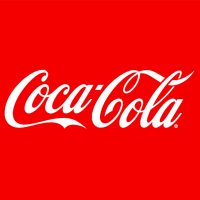# Coca-Cola Co NYSE:KOCoca-Cola Co
NYSE:KO
Price: 56.5 USD -0.88%
Updated:

-

-

## FAQ

### What is Discount Rate?

Discount rate reflects the opportunity cost of investment (i.e. the return that could be earned on investment with similar risk).

### Cost of Equity Calculation

Cost of Equity
-
Rf
-
Beta
-
ERP
-

The Cost of Equity for Coca-Cola Co (NYSE:KO) calculated via CAPM (Capital Asset Pricing Model) is -.

### WACC Calculation

WACC
-

Cost of Equity
-
Equity Weight
-

Cost of Debt
-
Debt Weight
-

The WACC for Coca-Cola Co (NYSE:KO) is -.